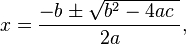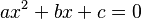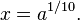# Algebraic solution facts for kids

Kids Encyclopedia Facts

An algebraic solution is an algebraic expression which is the solution of an algebraic equation in terms of the coefficients of the variables. It is found only by addition, subtraction, multiplication, division, and the extraction of roots (square roots, cube roots, etc.).

The most well-known example is the solution of the general quadratic equation.$x=\frac{-b \pm \sqrt {b^2-4ac\ }}{2a},$$ax^2 + bx + c =0\,$

(where a ≠ 0).

There is a more complicated solution for the general cubic equation and quartic equation. The Abel-Ruffini theorem states that the general quintic equation does not have an algebraic solution. This means that the general polynomial equation of degree n, for n ≥ 5, cannot be solved by using algebra. However, under certain conditions, we can get algebraic solutions; for example, the equation$x^{10} = a$ can be solved as$x=a^{1/10}.$In Spanish: Solución algebraica para niños